1、点击 IP Catalog，搜索 ila 核，双击 ILA 的 IP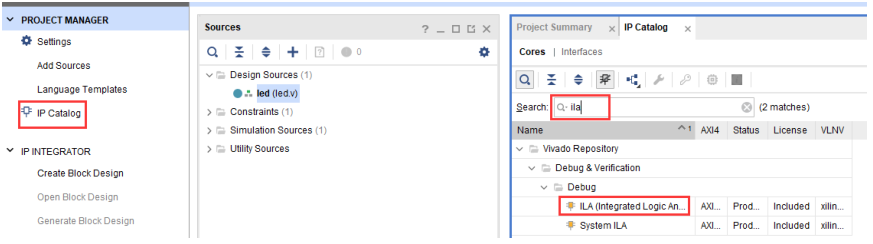module pl_led(
input sys_clk,
input rst_n,
output reg [3:0] led
);
parameter max_cnt = 32'd49999999;
reg [31:0] timer_cnt;

always @ (posedge sys_clk or negedge rst_n)
begin
if(!rst_n)
begin
timer_cnt <= 32'd0;
end
else
begin
if (timer_cnt < max_cnt) timer_cnt <= timer_cnt + 32'd1;
else timer_cnt <= 32'd0;
end
end

always @ (posedge sys_clk or negedge rst_n)
begin
if(!rst_n)
begin
led <= 4'b0000;
end
else
begin
if(timer_cnt == max_cnt) led <= ~led;
else led <= led;
end
end

endmodule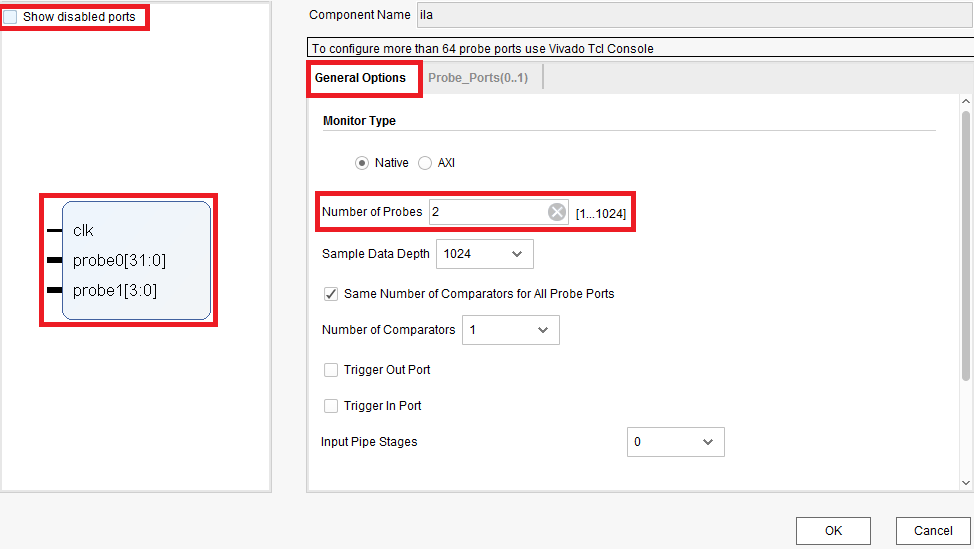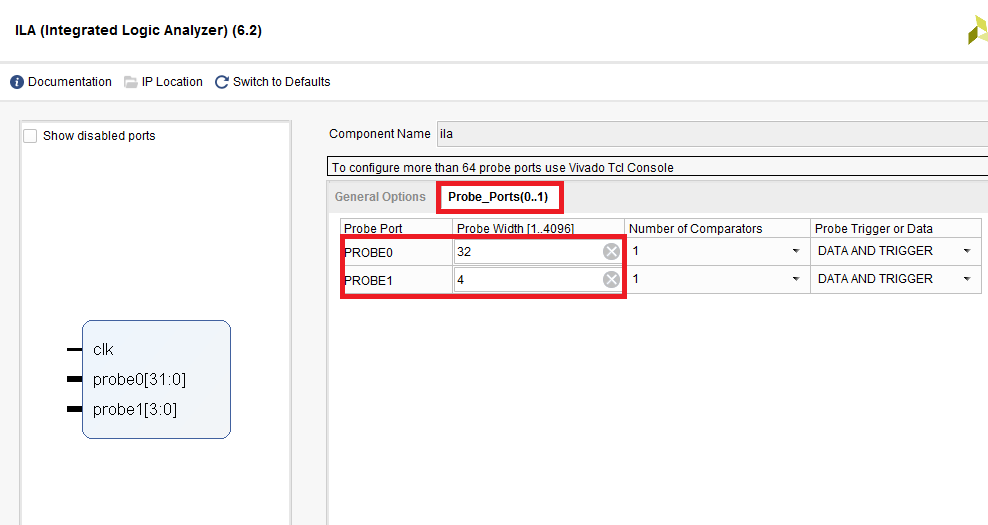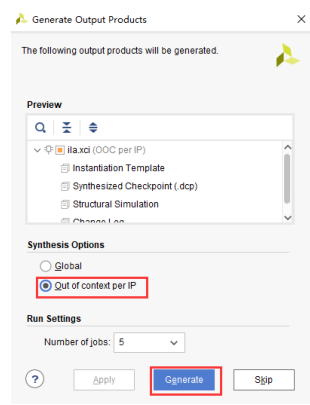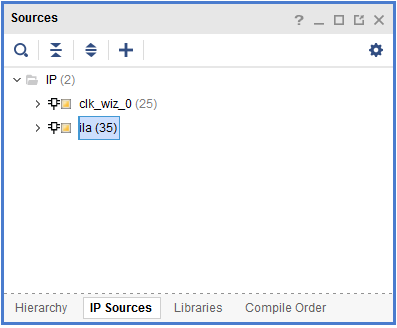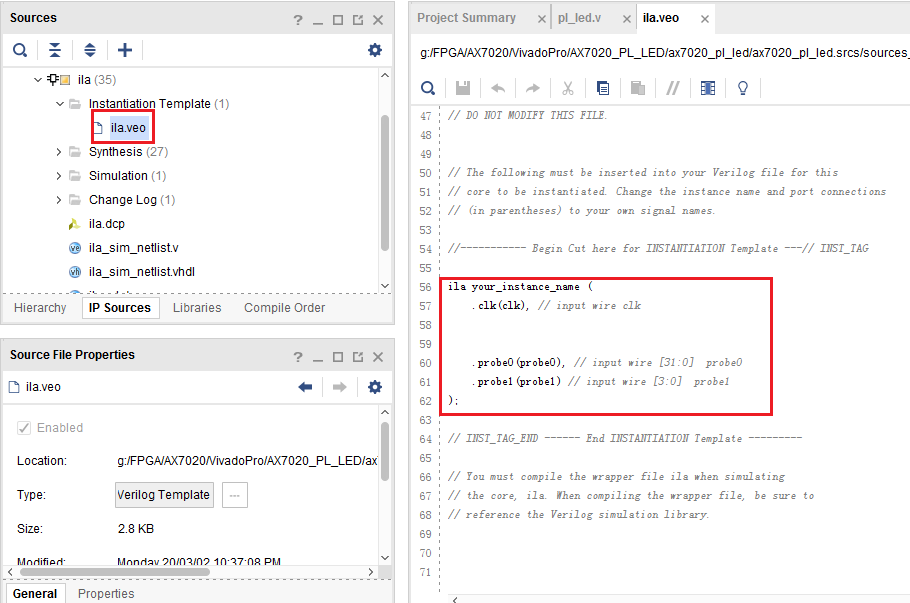module pl_led(
input sys_clk,
input rst_n,
output reg [3:0] led
);
parameter max_cnt = 32'd49999999;
reg [31:0] timer_cnt;

always @ (posedge sys_clk or negedge rst_n)
begin
if(!rst_n)
begin
timer_cnt <= 32'd0;
end
else
begin
if (timer_cnt < max_cnt) timer_cnt <= timer_cnt + 32'd1;
else timer_cnt <= 32'd0;
end
end

always @ (posedge sys_clk or negedge rst_n)
begin
if(!rst_n)
begin
led <= 4'b0000;
end
else
begin
if(timer_cnt == max_cnt) led <= ~led;
else led <= led;
end
end

// Instant ila in source code
ila ila_inst(
.clk(sys_clk),
.probe0(timer_cnt),
.probe1(led)
);

endmodule


OK，搞定，重新 Generate 生成 Bit Stream；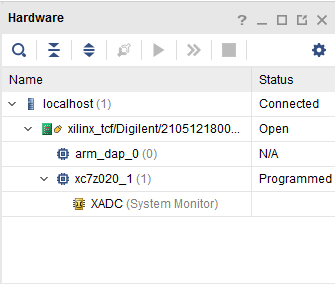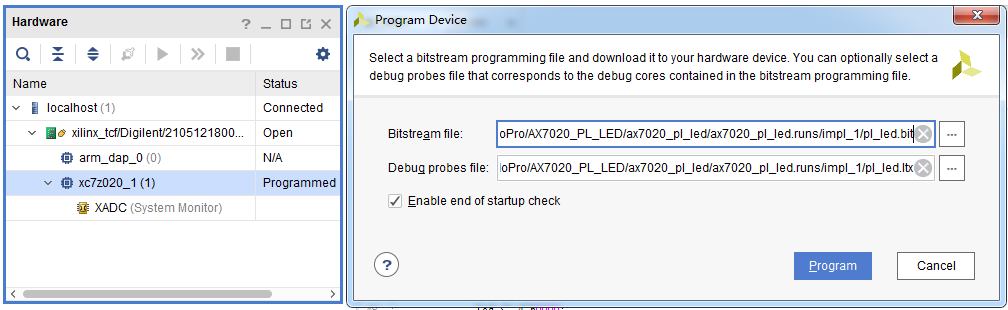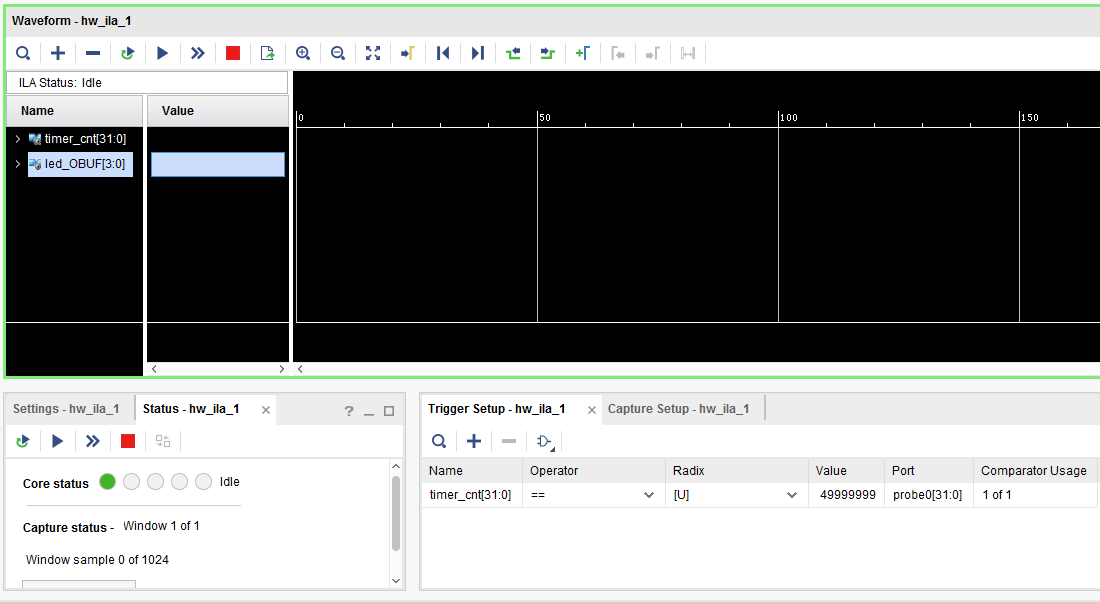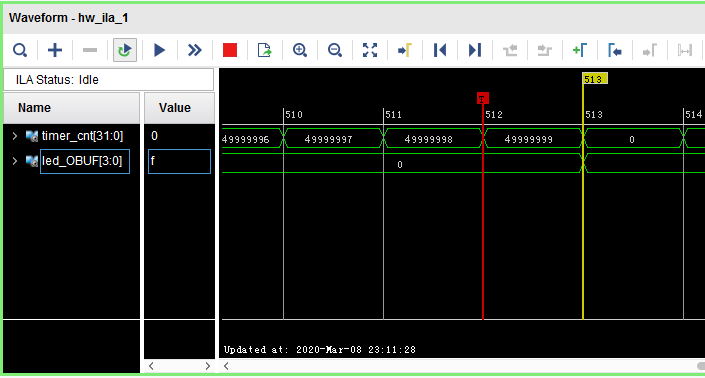11-071万+
07-0468410-151万+
03-221526
08-305750
11-052万+
06-031万+
01-041651
04-10580
07-03486
12-161万+
03-28718
04-133236
04-053978
04-251498